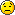Page 1 of 1

### conversion calculations

Posted: Tue Mar 23, 2021 1:57 pm
Calculate the molarity, normality and molality of a 32% H 2 CO 3 solution with a density of 1.18g / ml.### Re: conversion calculation

Posted: Tue Mar 23, 2021 2:10 pm
Calculate the mass of one 1l . 32% is the mass of the 100% carbonic acid. Convert this with molar mass to mole. The rest should be easy now

### Re: conversion calculations

Posted: Tue Mar 23, 2021 4:58 pm
mmmmmmm thank you

### Re: conversion calculations

Posted: Wed Mar 24, 2021 1:45 am
What is wrong, what you dont understand.

### Re: conversion calculations

Posted: Fri Mar 26, 2021 12:49 pm
wrong what'?

### Re: conversion calculations

Posted: Fri Mar 26, 2021 12:53 pm
I expect instead of mmmmmm, that you post your calculation or the result.

### Re: conversion calculations

Posted: Thu Apr 01, 2021 5:44 pm
Now calm because the mass is 7.57, the morality is 6.25 and the normality is 12.5
im doing well? :p

### Re: conversion calculations

Posted: Fri Apr 02, 2021 4:09 am
What is 7.57 eggs because of easter?

1 l= 1.18 kg
32% is 377,6 g = m

n =m/M 377,6 g/ 62 g/mol = 6,09 mol = Molarity
Normality is double = 12,18 mol

### Re: conversion calculations

Posted: Fri Apr 02, 2021 1:22 pm
but my teacher says me that im correct((

### Re: conversion calculations

Posted: Fri Apr 02, 2021 1:39 pm
Show the calculation